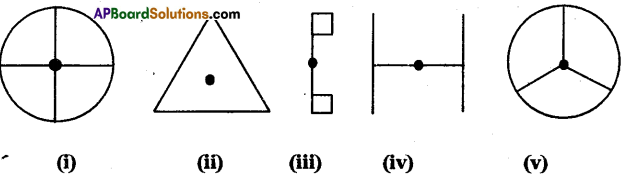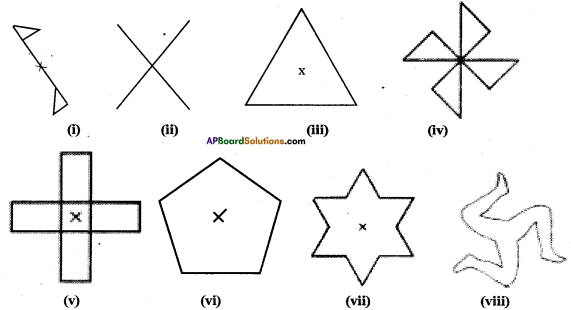AP State Syllabus AP Board 7th Class Maths Solutions Chapter 15 Symmetry Ex 3 Textbook Questions and Answers.

## AP State Syllabus 7th Class Maths Solutions 15th Lesson Symmetry Exercise 3Question 1.
Which of the following figures have rotational symmetry of order more than 1?Solution:
The above figure (i), (ii), (iii), (iv), (v) have rational symmetry of order more than 1.Question 2.
Give the order of rotational symmetry for each figure.Solution:
(i) Order 2
(ii) Order 4
(iii) Order 3
(iv) Order 4
(v) Order 4
(vi) Order 5
(vii) Order 6
(viii) Order 3